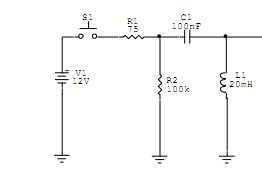# What are the initial conditions in this simple RCL circuit?So, let's assume the switch has been closed for a long time. The capacitor is charged to 12V, and the coil acts like a short-circuit.

Immediately after opening the switch, is it right to say the Voltage in the coil is 12V? My notes specify something along the lines of: the conditions at time 0+ must be the same as the conditions at time 0- to preserve continuity, because instantaneous variations are impossible. Are my notes wrong? Is the voltage in the coil at 0+ really 12V?

vk6kro
No, the capacitor has the 100 K resistor and the inductor effectively in series across it, so the majority of the voltage will appear across the resistor.

Really..? Doesn't that contradict the fact that V = L di/dt in the inductor? Isn't di/dt the biggest when the current goes from 0A to whatever it is right after that?

vk6kro
I did a simulation of this and there is a very brief negative going pulse of about 4.9 volts across the inductor when the switch is opened. This pulse is about 1μS wide.

The 100 K limits the current which is almost unchanged after the switch is opened. It gets 12 volts from the battery before the switch is opened and it gets 12 volts from the capacitor after the switch is opened.

After the initial pulse across the inductor, the voltage drops close to zero and the current in the inductor is about 120 μA which is due to the capacitor discharging via the 100 K resistor. This decreases with time.

Thanks, I was asking the question to find the initial conditions of the differential equation. I'm assuming that VL(0) is either 0 or 12V, which one is it and why?

NUCENG